JULIDE
Statistical Test
français | english
 Navigation If you click on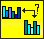, the following options appear :The statistical tool box is divided into three steps : 1. Create Groups : Compute mean and var images of a group of individual 2. Ttest Computation : Compute t-test between two groups 3. Ttest Visualisation : To visiualize t-test between two groups   1. Create Groups The fisrt step to compare groups of brain is to compute mean and var images of a group. To do so, use the following option :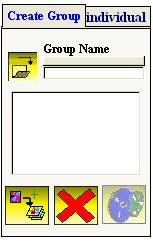First create a new directory for the mean and variance images as for exemple : Ttest/AC-OLP and give a name to the group, for example AC Then load images using the button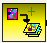then press the compute button :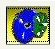Four images will be created : AC-MeanImage.mhd + AC-MeanImage.raw and AC-VarImage.mhd + AC-VarImage.raw A file which contains the number of individual in the groups is also created : AC-NumberOfIndi.txt   2. Ttest Then once you have computed the mean and var images of two groups you can compare them using the option ttest :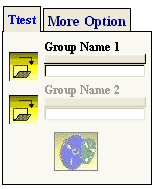First load the Mean image of group 1, for example AC-MeanImage.mhd Then load the Mean image of group 2, for example D1-MeanImage.mhd press the compute buttonat the end of the computation, you will be asked for the path and directory to save images. For example, create a new directory : Ttest/AC-OLP_vs_D1-OLP and called the image AC-OLP_vs_D1-OLP.mhd Three image are created : 1. Mean image of both groups : AC-OLP_vs_D1-OLP-MeanIm.mhd 2. Positive Result of ttest which means AC vs D1 : AC-OLP_vs_D1-OLP-Pos.mhd 3. Negative Result of ttest which means D1 vs AC : AC-OLP_vs_D1-OLP-Neg.mhd A file with the total number of individual is also created to determine the degree of freedom for the t-test visualisation.   3. Ttest visualisation If you want to visualize results of groups comparison, use the following option :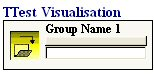Click on the load button, first load the mean image of both group. For example : AC-OLP_vs_D1-OLP-MeanIm.mhd then load the positive or negative ttest test for example AC-OLP_vs_D1-OLP-Neg.mhd. The following window appear :To change the p value use the p value window. For example instead of 0.05, use 0.02, press the ok button,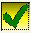, then you get :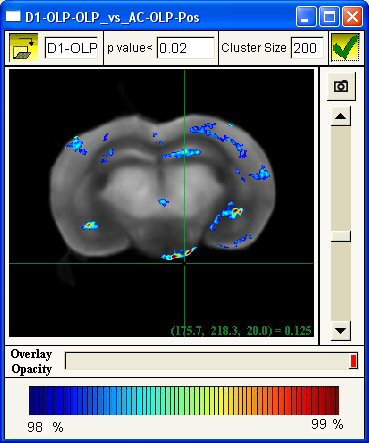It the same principle with the cluster size option, instead of 200 use 1000, then press the ok button,, then you get :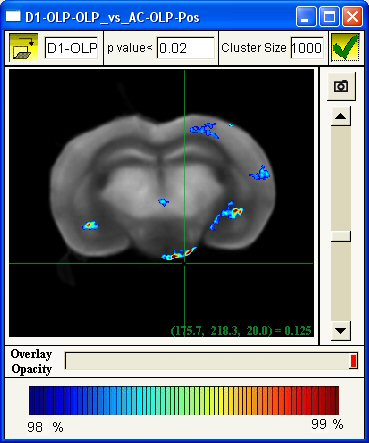Search